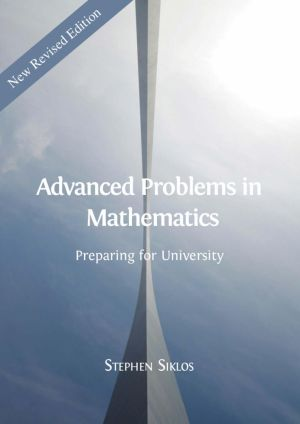## Preparing for University

by Stephen Siklos### Book Description

This new and expanded edition is intended to help candidates prepare for entrance examinations in mathematics and scientific subjects, including STEP (Sixth Term Examination Paper). STEP is an examination used by Cambridge Colleges for conditional offers in mathematics. They are also used by some other UK universities and many mathematics departments recommend that their applicants practice on the past papers even if they do not take the examination.

Advanced Problems in Mathematics bridges the gap between school and university mathematics, and prepares students for an undergraduate mathematics course. The questions analysed in this book are all based on past STEP questions and each question is followed by a comment and a full solution. The comments direct the reader's attention to key points and put the question in its true mathematical context. The solutions point students to the methodology required to address advanced mathematical problems critically and independently.

This book is a must read for any student wishing to apply to scientific subjects at university level and for anyone interested in advanced mathematics.

Problem 1
An integer equation
Problem 2
Partitions of 10 and 20
Problem 3
Mathematical deduction
Problem 4
Divisibility
Problem 5
The modulus function
Problem 6
The regular Reuleaux heptagon
Problem 7
Chain of equations
Problem 8
Trig. equations
Problem 9
Integration by substitution
Problem 10
True or false
Problem 11
Egyptian fractions
Problem 12
Maximising with constraints
Problem 13
Binomial expansion
Problem 14
Sketching subsets of the plane
Problem 15
More sketching subsets of the plane
Problem 16
Non-linear simultaneous equations
Problem 17
Inequalities
Problem 18
Inequalities from cubics
Problem 19
Logarithms
Problem 20
Cosmological models
Problem 21
Melting snowballs
Problem 22
Gregory's series
Problem 23
Intersection of ellipses
Problem 24
Sketching x^m(1 - x)^n
Problem 25
Inequalities by area estimates
Problem 26
Simultaneous integral equations
Problem 27
Relation between coefficients of quartic for real roots
Problem 28
Fermat numbers
Problem 29
Telescoping series
Problem 30
Integer solutions of cubics
Problem 31
The harmonic series
Problem 32
Integration by substitution
Problem 33
More curve sketching
Problem 34
Trig. sum
Problem 35
Roots of a cubic equation
Problem 36
Root counting
Problem 37
Irrationality of e
Problem 38
Discontinuous integrands
Problem 39
A difficult integral
Problem 40
Estimating the value of an integral
Problem 41
Integrating the modulus function
Problem 42
Geometry
Problem 43
The t substitution
Problem 44
A differential-difference equation
Problem 45
Lagrange's identity
Problem 46
Bernoulli polynomials
Problem 47
Vector geometry
Problem 48
Solving a quartic
Problem 49
Areas and volumes
Problem 50
More curve sketching
Problem 51
Spherical loaf
Problem 52
Snowploughing
Problem 53
Tortoise and hare
Problem 54
How did the chicken cross the road?
Problem 55
Hank's gold mine
Problem 56
A chocolate orange
Problem 57
Lorry on bend
Problem 58
Fielding
Problem 59
Equilibrium of rod of non-uniform density
Problem 60
Problem 61
Kinematics of rotating target
Problem 62
Particle on wedge
Problem 63
Sphere on step
Problem 64
Elastic band on cylinder
Problem 65
A knock-out tournament
Problem 66
Harry the calculating horse
Problem 67
PIN guessing
Problem 68
Breaking plates
Problem 69
Lottery
Problem 70
Bodies in the fridge
Problem 71
Choosing keys
Problem 72
Commuting by train
Problem 73
Collecting voles
Problem 74
Breaking a stick
Problem 75

### Book Details

Subject
Science and Mathematics
Publisher
Open Book Publishers
Published
2019
Pages
186
Edition
1
Language
English
ISBN13
9781783747764
ISBN10
1783747765
ISBN13 Digital
9781783747771
ISBN10 Digital
1783747773
PDF Size
2.0 MB### Related BooksThe purpose of this Open Access compendium, written by experienced researchers in mathematics education, is to serve as a resource for early career researchers in furthering their knowledge of the state of the field and disseminating their research through publishing. To accomplish this, the book is split into four sections: Empirical Methods, Impo...This book is the first major publication on the topic of "Interdisciplinary Mathematics Education" and arose from the work of the first International Topic Study Group of the same name at the ICME-13 conference in Hamburg in 2016. It offers extensive theoretical insights, empirical research, and practitioner accounts of interdisciplinary ...This engaging free book discusses how a values and valuing perspective can facilitate a more effective mathematics pedagogical experience, and allows readers to explore multiple applications of the values perspective across different education systems. It also clearly shows that teaching mathematics involves not only reasoning and feelings, but als...Classic Computer Science Problems in Python deepens your knowledge of problem solving techniques from the realm of computer science by challenging you with time-tested scenarios, exercises, and algorithms. As you work through examples in search, clustering, graphs, and more, you'll remember important things you've forgotten and discover classic sol...This open access report explores the nature and extent of students' misconceptions and misunderstandings related to core concepts in physics and mathematics and physics across grades four, eight and 12. Twenty years of data from the IEA's Trends in International Mathematics and Science Study (TIMSS) and TIMSS Advanced assessments are analyzed, spec...This book examines the kinds of transitions that have been studied in mathematics education research. It defines transition as a process of change, and describes learning in an educational context as a transition process. The book focuses on research in the area of mathematics education, and starts out with a literature review, describing the epist...